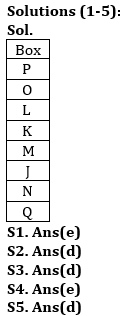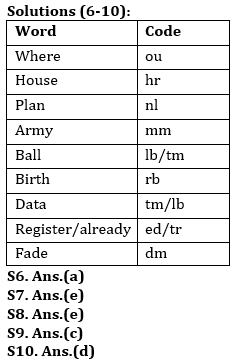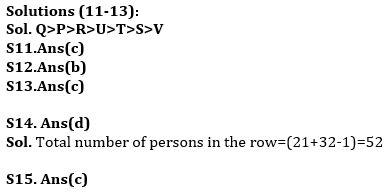Latest Banking jobs   »   Reasoning Ability Quiz For ESIC- UDC,...

# Reasoning Ability Quiz For ESIC- UDC, Steno, MTS Prelims 2022- 4th January

Directions (1-5): Study the information carefully and answer the questions given below.
There are eight boxes J, K, L, M, N, O, P and Q are placed one above another. Two boxes are placed between Box K and Box N. Box Q is placed immediately below Box N. One box is placed between Box P and Box L. Box M is placed one of the above place of N and one of the below place of Box O but not immediate above or below. Box J and Box O is not placed above Box P.

Q1. Which of the following box is placed just below box L?
(a) N
(b) Q
(c) M
(d) O
(e) None of these

Q2. Which of the following box is placed just above box N?
(a) Q
(b) L
(c) M
(d) J
(e) None of these

Q3. How many boxes are placed between box O and box N?
(a) Three
(b) Two
(c) One
(d) Four
(e) None

Q4. What is the position of box M?
(a) Third from the top
(b) Second from the top
(c) Third from the bottom
(d) Second from the bottom
(e) None of these

Q5. Four of the following five are alike in certain way based from a group, find the one that does not belong to that group?
(a) P-L
(b) O-K
(c) N-M
(d) L-N
(e) Q-J

Directions (6-10): Study the following information carefully and answer the questions given below:
In a certain code language
‘where house plan army’ is written as ‘nl am hr ou’,
‘ball house birth data’ is written as ‘tm rb lb hr’,
‘army plan register already’ is written as ‘ed nl am tr’,
‘fade plan data ball’ is written as ‘tm lb nl dm’.

Q6. What is the code for ‘Fade’?
(a) dm
(b) nl
(c) lb
(d) tm
(e) None of these

Q7. Which of the following may be the code for ‘already house plan’?
(a) ed hr rb
(b) tr nl hr
(c) rb hr tr
(d) ed nl hr
(e) Either (b) or (d)

Q8. What is the code for ‘Register’?
(a) tr
(b) am
(c) nl
(d) ed
(e) Can’t be determined

Q9. What does ‘rb’ stand for?
(a) House
(b) Ball
(c) Birth
(d) Data
(e) None of these

Q10. What is the code for ‘Plan’?
(a) am
(b) hr
(c) lb
(d) nl
(e) Can’t be determined

Directions (11-13): Study the information carefully and answer the questions given below.
Seven persons i.e. P, Q, R, S, T, U and V are scoring different marks in an examination. P score more marks than R. T score more marks than V and less than U. P does not score the highest. S score less than T and more than only one person. R score more than U.

Q11. Who among the following person score highest marks?
(a) U
(b) R
(c) Q
(d) T
(e) None of these

Q12. How many persons score less than V?
(a) One
(b) None
(c) Two
(d) Three
(e) Five

Q13. Who among the following person score third highest?
(a) V
(b) P
(c) R
(d) T
(e) None of these

Q14. Anchal is 30th from the left end of a row and Teena is 21st from the right end of row. If they interchanged their positions then anchal ranks become 32 from left end. Find total number of persons in the row?
(a) 51
(b) 47
(c) 58
(d) 52
(e) None of these

Q15. Demon’s ranked 24th from the top in a class of 64 students. What is the rank of demon from the bottom?
(a) 40
(b) 32
(c) 41
(d) 29
(e) None of these

Solutions#### Congratulations!Union Budget 2023-24: Free PDF